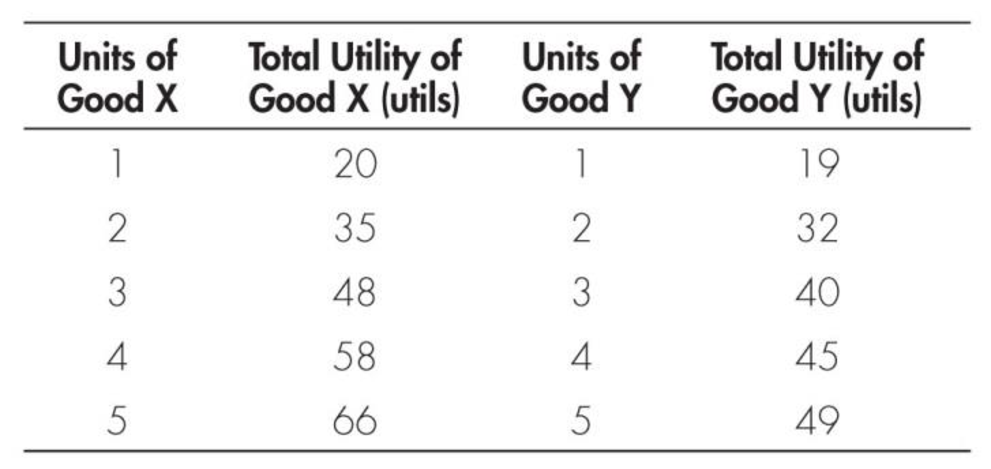Chapter 7, Problem 4WNG

Chapter
Section
Textbook Problem

Use the following table to answer Questions 4 and 5:4. If George spends $5 (total) a week on good X and good Y, and if the price of each good is$1 per unit, then how many units of each good does he purchase to maximize utility?

To determine

Identify the maximization of utility.

Explanation

Marginal utility (MU) can be calculated using the following formula:

Marginal utility=Change in total utilityChange in quantity (1)

Since the value of the total utility of the 2 units of X is 35 and the total utility of 1unit is 20, the marginal utility of the 2 units can be calculated using Equation -1 as follows:

Marginal utility=35utils20utils21<

Still sussing out bartleby?

Check out a sample textbook solution.

See a sample solution

The Solution to Your Study Problems

Bartleby provides explanations to thousands of textbook problems written by our experts, many with advanced degrees!

Get Started

Find more solutions based on key concepts